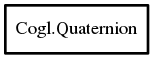# Quaternion

## Object Hierarchy:## Description:

[ Compact ]
public class Quaternion

A quaternion is comprised of a scalar component and a 3D vector component.

The scalar component is normally referred to as w and the vector might either be referred to as v or a (for axis) or expanded with the individual components: (x, y, z) A full quaternion would then be written as <literal>[w (x, y, z)]</literal>.

Quaternions can be considered to represent an axis and angle pair although sadly these numbers are buried somewhat under some maths...

For the curious you can see here that a given axis (a) and angle (𝜃) pair are represented in a quaternion as follows:

`[w=cos(𝜃/2) ( x=sin(𝜃/2)*a.x, y=sin(𝜃/2)*a.y, z=sin(𝜃/2)*a.x )]`
rnions: When using Quaternions to represent spatial orientations for 3D graphics it's always assumed you have a unit quaternion. The magnitude of a quaternion is defined as:
`sqrt (w² + x² + y² + z²)`
a unit quaternion satisfies this equation:
`w² + x² + y² + z² = 1`
ankfully most of the time we don't actually have to worry about the maths that goes on behind the scenes but if you are curious to learn more here are some external references:

<itemizedlist> <listitem> <ulink url="http://mathworld.wolfram.com/Quaternion.html"/> </listitem> <listitem > <ulink url="http://www.gamedev.net/reference/articles/article1095.asp"/> </listitem> <listitem> <ulink url="http://www.cprogramming.com/tutorial/3d/quaternions.html"/> </listitem> <listitem> <ulink url="http://www.isner.com/tutorials/quatSpells/quaternion_spells_12.htm"/> </listitem> <listitem> 3D Maths Primer for Graphics and Game Development ISBN-10: 1556229119 </listitem> <listitem> <ulink url="http://www.cs.caltech.edu/courses/cs171/quatut.pdf"/> </listitem> <listitem> <ulink url="http://www.j3d.org/matrix_faq/matrfaq_latest.html#Q56"/> </listitem> </itemizedlist>

Namespace: Cogl
Package: doc